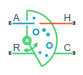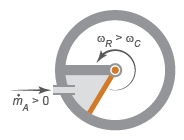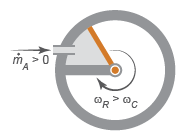# Rotational Mechanical Converter (2P)

Interface between two-phase fluid and mechanical rotational networks

## Library

Two-Phase Fluid/Elements

•## Description

The Rotational Mechanical Converter (2P) block models an interface between two-phase fluid and mechanical rotational networks. The interface converts pressure in the fluid network into torque in the mechanical rotational network and vice versa.

This block enables you to model a rotary actuator powered by a two-phase fluid system. It does not, however, account for inertia, friction, or hard stops, common in rotary actuators. You can model these effects separately using Simscape blocks such as Inertia, Rotational Friction, and Rotational Hard Stop.

Port A represents the inlet through which fluid enters and exits the converter. Ports C and R represent the converter casing and moving interface, respectively. Port H represents the wall through which the converter exchanges heat with its surroundings.

### Torque Direction

The torque direction depends on the mechanical orientation of the converter. If the Mechanical Orientation parameter is positive, then a positive flow rate through the inlet tends to rotate the moving interface in the positive direction relative to the converter casing.

Positive Mechanical OrientationIf the Mechanical Orientation parameter is negative, then a positive mass flow rate through the inlet tends to rotate the moving interface in the negative direction relative to the converter casing.

Negative Mechanical Orientation### Flow and Thermal Resistances

The flow resistance between port A and the converter interior is assumed negligible. Pressure losses between the two is approximately zero. The pressure at port A is therefore equal to that in the converter:

`${p}_{A}={p}_{I},$`

where:

• pA is the pressure at port A.

• pI is the pressure in the converter.

Similarly, the thermal resistance between port H and the converter interior is assumed negligible. The temperature gradient between the two is approximately zero. The temperature at port H is therefore equal to that in the converter:

`${T}_{H}={T}_{I},$`

where:

• TH is the temperature at port H.

• TI is the temperature in the converter.

### Fluid Volume

The volume of fluid in the converter is the sum of the dead and displaced fluid volumes. The dead volume is the amount of fluid left in the converter at a zero interface angle. This volume enables you to model the effects of dynamic compressibility and thermal capacity even when the interface is in its zero position.

The displacement volume is the amount of fluid added to the converter due to rotation of the moving interface. This volume increases with the interface angle. The total volume in the converter as a function of the interface angle is

`$V={V}_{dead}+{D}_{vol}{\theta }_{int}{ϵ}_{or},$`

where:

• V is the total volume of fluid in the converter.

• Dvol is the displaced fluid volume per unit rotation of the interface.

• θint is the rotation angle of the moving interface.

• or is the mechanical orientation of the converter (`1` if increase in fluid pressure causes positive rotation of R relative to C, `-1` if increase in fluid pressure causes negative rotation of R relative to C).

If you connect the converter to a Multibody joint, use the physical signal input port q to specify the rotation of port R relative to port C. Otherwise, the block calculates the interface rotation from relative port angular velocities, according to the block equations. The interface rotation is zero when the fluid volume is equal to the dead volume. Then, depending on the Mechanical orientation parameter value:

• If ```Pressure at A causes positive rotation of R relative to C```, the interface rotation increases when the fluid volume increases from dead volume.

• If ```Pressure at A causes negative rotation of R relative to C```, the interface rotation decreases when the fluid volume increases from dead volume.

### Force Balance

At equilibrium, the internal pressure in the converter counteracts the external pressure of its surroundings and the torque exerted by the mechanical network on the moving interface. This torque is the reverse of that applied by the fluid network. The torque balance in the converter is therefore

`${p}_{I}{D}_{vol}={p}_{atm}{D}_{vol}-{t}_{int}{ϵ}_{or},$`

where:

• patm is the environmental pressure outside the converter.

• tint is the magnitude of the torque exerted by the fluid network on the moving interface.

### Energy Balance

The total energy in the converter can change due to energy flow through the inlet, heat flow through the converter wall, and work done on the mechanical network. The energy flow rate, given by the energy conservation equation, is therefore

`$\stackrel{˙}{E}={\varphi }_{A}+{\varphi }_{H}-{p}_{I}{D}_{vol}{\stackrel{˙}{\theta }}_{int}{ϵ}_{or},$`

where:

• E is the total energy of the fluid in the converter.

• ϕA is the energy flow rate into the converter through port A.

• ϕH is the heat flow rate into the converter through port H.

Taking the fluid kinetic energy in the converter to be negligible, the total energy of the fluid reduces to:

`$E=M{u}_{I},$`

where:

• M is the fluid mass in the converter.

• uI is the specific internal energy of the fluid in the converter.

### Mass Balance

The fluid mass in the converter can change due to flow through the inlet, represented by port A. The mass flow rate, given by the mass conservation equation, is therefore

`$\stackrel{˙}{M}={\stackrel{˙}{m}}_{A},$`

where:

• ${\stackrel{˙}{m}}_{A}$ is the mass flow rate into the converter through port A.

A change in fluid mass can accompany a change in fluid volume, due to rotation of the moving interface. It can also accompany a change in mass density, due to an evolving pressure or specific internal energy in the converter. The mass rate of change in the converter is then

`$\stackrel{˙}{M}=\left[{\left(\frac{\partial \rho }{\partial p}\right)}_{u}{\stackrel{˙}{p}}_{I}+{\left(\frac{\partial \rho }{\partial u}\right)}_{p}{\stackrel{˙}{u}}_{I}\right]V+\frac{{D}_{vol}{\stackrel{˙}{\theta }}_{int}{ϵ}_{or}}{{v}_{I}},$`

where:

• ${\left(\frac{\partial \rho }{\partial p}\right)}_{u}$ is the partial derivative of density with respect to pressure at constant specific internal energy.

• ${\left(\frac{\partial \rho }{\partial u}\right)}_{p}$ is the partial derivative of density with respect to specific internal energy at constant pressure.

• vI is the specific volume of the fluid in the converter.

The block blends the density partial derivatives of the various domains using a cubic polynomial function. At a vapor quality of 0–0.1, this function blends the derivatives of the subcooled liquid and two-phase mixture domains. At a vapor quality of 0.9–1, it blends those of the two-phase mixture and superheated vapor domains.

The smoothed density partial derivatives introduce into the original mass conservation equation undesirable numerical errors. To correct for these errors, the block adds the correction term

`${ϵ}_{M}=\frac{M-V/{v}_{I}}{\tau },$`

where:

• M is the correction term.

• τ is the phase-change time constant—the characteristic duration of a phase change event. This constant ensures that phase changes do not occur instantaneously, effectively introducing a time lag whenever they occur.

The final form of the mass conservation equation is

`$\left[{\left(\frac{\partial \rho }{\partial p}\right)}_{u}{\stackrel{˙}{p}}_{I}+{\left(\frac{\partial \rho }{\partial u}\right)}_{p}{\stackrel{˙}{u}}_{I}\right]V+\frac{{D}_{vol}{\stackrel{˙}{\theta }}_{int}{ϵ}_{or}}{{v}_{I}}={\stackrel{˙}{m}}_{A}+{ϵ}_{M}.$`

The block uses this equation to calculate the internal pressure in the converter given the mass flow rate through the inlet.

## Assumptions and Limitations

• The converter walls are rigid. They do not deform under pressure.

• The flow resistance between port A and the converter interior is negligible. The pressure is the same at port A and in the converter interior.

• The thermal resistance between port H and the converter interior is negligible. The temperature is the same at port H and in the converter interior.

• The moving interface is perfectly sealed. No fluid leaks across the interface.

• Mechanical effects such as hard stops, inertia, and friction, are ignored.

## Parameters

### Parameters Tab

Mechanical orientation

Alignment of the moving interface with respect to the volume of fluid in the converter:

• ```Pressure at A causes positive rotation of R relative to C``` — Increase in the fluid volume results in a positive rotation of port R relative to port C.

• ```Pressure at A causes negative rotation of R relative to C``` — Increase in the fluid volume results in a negative rotation of port R relative to port C.

Interface rotation

Select method to determine rotation of port R relative to port C:

• ```Calculate from velocity of port R relative to port C``` — Calculate rotation from relative port velocities, based on the block equations. This is the default method.

• ```Provide input signal from Multibody joint``` — Enable the input physical signal port q to pass the rotation information from a Multibody joint. Use this method only when you connect the converter to a Multibody joint by using a Rotational Multibody Interface block. For more information, see How to Pass Position Information.

Initial interface rotation

Angle of the moving interface at the start of simulation. A zero angle corresponds to a total fluid volume in the converter equal to the specified dead volume. The default value is `0` rad.

• If Mechanical orientation is ```Pressure at A causes positive rotation of R relative to C```, the parameter value must be greater than or equal to 0.

• If Mechanical orientation is ```Pressure at A causes negative rotation of R relative to C```, the parameter value must be less than or equal to 0.

This parameter is enabled when Interface rotation is set to ```Calculate from velocity of port R relative to port C```.

Interface volume displacement

Displaced fluid volume per unit rotation of the moving interface. The default value is `0.01 m^3`/rad.

Volume of fluid left in the converter when the interface angle is zero. The dead volume enables the block to account for mass and energy storage in the converter even at a zero interface angle. The default value is `1e-5` m^3.

Cross-sectional area at port A

Flow area of the converter inlet, represented by port A. Pressure losses due to changes in flow area inside the converter are ignored. The default value is `0.01` m^2.

Environment pressure specification

Pressure characteristics of the surrounding environment. Select ```Atmospheric pressure``` to set the environment pressure to the atmospheric pressure specified in the Two-Phase Fluid Properties (2P) block. Select `Specified pressure` to set the environment pressure to a different value. The default setting is `Atmospheric pressure`.

Environment pressure

Absolute pressure of the surrounding environment. The environment pressure acts against the internal pressure of the converter and affects the motion of the converter shaft. This parameter is active only when the Environment pressure specification parameter is set to `Specified pressure`. The default value, `0.101325` MPa, corresponds to atmospheric pressure at mean sea level.

### Effects and Initial Conditions Tab

Initial fluid energy specification

Thermodynamic variable in terms of which to define the initial conditions of the component. The default setting is `Temperature`.

Initial pressure

Pressure in the chamber at the start of simulation, specified against absolute zero. The default value is `0.101325` MPa.

Initial temperature

Temperature in the chamber at the start of simulation, specified against absolute zero. This parameter is active when the Initial fluid energy specification option is set to `Temperature`. The default value is `293.15` K.

Initial vapor quality

Mass fraction of vapor in the chamber at the start of simulation. This parameter is active when the Initial fluid energy specification option is set to ```Vapor quality```. The default value is `0.5`.

Initial vapor void fraction

Volume fraction of vapor in the chamber at the start of simulation. This parameter is active when the Initial fluid energy specification option is set to ```Vapor void fraction```. The default value is `0.5`.

Initial specific enthalpy

Specific enthalpy of the fluid in the chamber at the start of simulation. This parameter is active when the Initial fluid energy specification option is set to `Specific enthalpy`. The default value is `1500` kJ/kg.

Initial specific internal energy

Specific internal energy of the fluid in the chamber at the start of simulation. This parameter is active when the Initial fluid energy specification option is set to `Specific internal energy`. The default value is `1500` kJ/kg.

Phase change time constant

Characteristic duration of a phase-change event. This constant introduces a time lag into the transition between phases. The default value is `0.1` s.

## Ports

The block has the following ports:

`A`

Two-phase fluid conserving port associated with the converter inlet.

`H`

Thermal conserving port representing the converter surface through which heat exchange occurs.

`R`

Mechanical rotational conserving port associated with the converter rotor.

`C`

Mechanical rotational conserving port associated with the converter case.

`P`

Physical signal input port that passes the position information from a Simscape™ Multibody™ joint. Connect this port to the position sensing port q of the joint. For more information, see Connecting Simscape Networks to Simscape Multibody Joints. To enable this port, set the Interface rotation parameter to ```Provide input signal from Multibody joint```.

## Version History

Introduced in R2015b